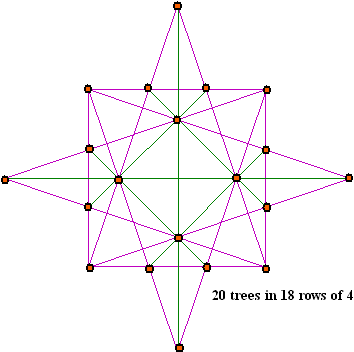# Magic Star Updates

This page, started in March, 2005, will contain material added to this site or links to sub-pages of such material.## Index to new material

### Simon Whitechapel

Emails starting in 2001. Simon presents solutions for pattern A of magic stars from 15 to 100.

### Jon Wharf

Emails starting in 2003. Jon confirms the total solution count for all orders and patterns from 6 to11, and provides the total solution count all order 12 patterns. He also supplies some solutions for all patterns of orders 13 and 14.

### Andrew Howroyd

First contacted me in February, 2005. He also confirms all total solution counts and investigated permutations  between patterns of orders 10 and 11.

### Tree Planting problems

A common recreational mathematics puzzle. here it is limited to star shaped patterns.

### A Fractional Star

This novelty star consists of proper fractions instead of integers.

More short articles will appear on this page as they become available.### Tree Planting problems

A type of puzzle common in recreational mathematics is referred to as ætree plantingÆ. They are so called because they are usually presented as
ōA farmer wished to plant x trees in y rows of z trees each. How should he do this.ö

The magic stars may be considered to be tree planting solutions ,with order 5 being 10 trees in 5 rows of 4; order 6 being 12 trees in 6 rows of 4, etc.
My order 5 page shows the 6 patterns possible for 10 trees in 5 rows of 4. One is the magic pentagram, the other five have the same numbers suitable placed so they are magic also. My Polygons and Graphs page has much more on tree-planting problems.

Here I will present some more complicated star shaped patterns. Interested readers may wish to try assigning numbers so all lines sum the same.This diagram is the solution to problem 21 in DudeneyÆs Cantabury Puzzles (page 175). It has 16 cells to place numbers so ideally would use the numbers 1 to 16 arranged so the 15 lines all sum to the same  value.  Is this possible?This stylized order 8 contain 4 more spots for numbers. Here we would use the numbers 1 to 20 arranged so all 18 rows sum correctly.
 I have not been able to design a star-like pattern where the number of trees is less then the number of lines. However, the first diagram can be modified to come close, by introducing a third 5 point star to give 20 trees in 20 row of 4.### A Fractional Star

 This novelty star was found in a grade five exercise booklet (1)   Add the 4 fractions in each of the six lines. Are the six sums the same? Add the 3 fractions in each of the two big triangles. Are the two sums the same? Add the 3 fractions in each of the six small triangles. Are the six sums the same? (1) Harold D. Larson,  Magic Squares, Circles, Stars: Grade 5., 16pp booklet published in 1956.Harvey Heinz   harveyheinz@shaw.ca
This page last updated January 27, 2009
Copyright ® 2005 by Harvey D. Heinz